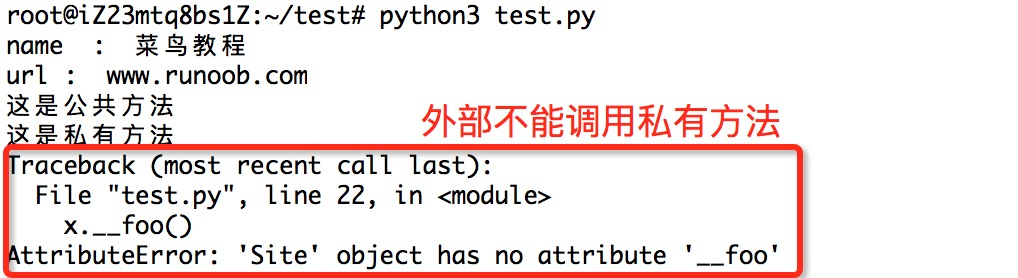# Python3基础之Python3面向对象

Python3.X作者：顾小北日期：2021-04-28点击：65

Python从设计之初就已经是一门面向对象的语言，正因为如此，在Python中创建一个类和对象是很容易的。本章节我们将详细介绍Python的面向对象编程。

## 面向对象技术简介

• 类(Class): 用来描述具有相同的属性和方法的对象的集合。它定义了该集合中每个对象所共有的属性和方法。对象是类的实例。
• 方法：类中定义的函数。
• 类变量：类变量在整个实例化的对象中是公用的。类变量定义在类中且在函数体之外。类变量通常不作为实例变量使用。
• 数据成员：类变量或者实例变量用于处理类及其实例对象的相关的数据。
• 方法重写：如果从父类继承的方法不能满足子类的需求，可以对其进行改写，这个过程叫方法的覆盖（override），也称为方法的重写。
• 局部变量：定义在方法中的变量，只作用于当前实例的类。
• 实例变量：在类的声明中，属性是用变量来表示的，这种变量就称为实例变量，实例变量就是一个用 self 修饰的变量。
• 继承：即一个派生类（derived class）继承基类（base class）的字段和方法。继承也允许把一个派生类的对象作为一个基类对象对待。例如，有这样一个设计：一个Dog类型的对象派生自Animal类，这是模拟"是一个（is-a）"关系（例图，Dog是一个Animal）。
• 实例化：创建一个类的实例，类的具体对象。
• 对象：通过类定义的数据结构实例。对象包括两个数据成员（类变量和实例变量）和方法。

Python中的类提供了面向对象编程的所有基本功能：类的继承机制允许多个基类，派生类可以覆盖基类中的任何方法，方法中可以调用基类中的同名方法。

## 类定义

class ClassName: <statement-1> . . . <statement-N>

## 实例(Python 3.0+)

#!/usr/bin/python3 class MyClass: """一个简单的类实例""" i = 12345 def f(self): return 'hello world' # 实例化类 x = MyClass() # 访问类的属性和方法 print("MyClass 类的属性 i 为：", x.i) print("MyClass 类的方法 f 输出为：", x.f())

```MyClass 类的属性 i 为： 12345
MyClass 类的方法 f 输出为： hello world
```

def __init__(self): self.data = []

```x = MyClass()
```

## 实例(Python 3.0+)

#!/usr/bin/python3 class Complex: def __init__(self, realpart, imagpart): self.r = realpart self.i = imagpart x = Complex(3.0, -4.5) print(x.r, x.i) # 输出结果：3.0 -4.5

### self代表类的实例，而非类

class Test: def prt(self): print(self) print(self.__class__) t = Test() t.prt()

```<__main__.Test instance at 0x100771878>
__main__.Test
```

self 不是 python 关键字，我们把他换成 runoob 也是可以正常执行的:

class Test: def prt(runoob): print(runoob) print(runoob.__class__) t = Test() t.prt()

```<__main__.Test instance at 0x100771878>
__main__.Test
```

## 实例(Python 3.0+)

#!/usr/bin/python3 #类定义 class people: #定义基本属性 name = '' age = 0 #定义私有属性,私有属性在类外部无法直接进行访问 __weight = 0 #定义构造方法 def __init__(self,n,a,w): self.name = n self.age = a self.__weight = w def speak(self): print("%s 说: 我 %d 岁。" %(self.name,self.age)) # 实例化类 p = people('runoob',10,30) p.speak()

```runoob 说: 我 10 岁。
```

## 继承

Python 同样支持类的继承，如果一种语言不支持继承，类就没有什么意义。派生类的定义如下所示:

class DerivedClassName(BaseClassName): <statement-1> . . . <statement-N>

BaseClassName（实例中的基类名）必须与派生类定义在一个作用域内。除了类，还可以用表达式，基类定义在另一个模块中时这一点非常有用:

```class DerivedClassName(modname.BaseClassName):
```

## 实例(Python 3.0+)

#!/usr/bin/python3 #类定义 class people: #定义基本属性 name = '' age = 0 #定义私有属性,私有属性在类外部无法直接进行访问 __weight = 0 #定义构造方法 def __init__(self,n,a,w): self.name = n self.age = a self.__weight = w def speak(self): print("%s 说: 我 %d 岁。" %(self.name,self.age)) #单继承示例 class student(people): grade = '' def __init__(self,n,a,w,g): #调用父类的构函 people.__init__(self,n,a,w) self.grade = g #覆写父类的方法 def speak(self): print("%s 说: 我 %d 岁了，我在读 %d 年级"%(self.name,self.age,self.grade)) s = student('ken',10,60,3) s.speak()

```ken 说: 我 10 岁了，我在读 3 年级
```

## 多继承

Python同样有限的支持多继承形式。多继承的类定义形如下例:

class DerivedClassName(Base1, Base2, Base3): <statement-1> . . . <statement-N>

## 实例(Python 3.0+)

#!/usr/bin/python3 #类定义 class people: #定义基本属性 name = '' age = 0 #定义私有属性,私有属性在类外部无法直接进行访问 __weight = 0 #定义构造方法 def __init__(self,n,a,w): self.name = n self.age = a self.__weight = w def speak(self): print("%s 说: 我 %d 岁。" %(self.name,self.age)) #单继承示例 class student(people): grade = '' def __init__(self,n,a,w,g): #调用父类的构函 people.__init__(self,n,a,w) self.grade = g #覆写父类的方法 def speak(self): print("%s 说: 我 %d 岁了，我在读 %d 年级"%(self.name,self.age,self.grade)) #另一个类，多重继承之前的准备 class speaker(): topic = '' name = '' def __init__(self,n,t): self.name = n self.topic = t def speak(self): print("我叫 %s，我是一个演说家，我演讲的主题是 %s"%(self.name,self.topic)) #多重继承 class sample(speaker,student): a ='' def __init__(self,n,a,w,g,t): student.__init__(self,n,a,w,g) speaker.__init__(self,n,t) test = sample("Tim",25,80,4,"Python") test.speak() #方法名同，默认调用的是在括号中排前地父类的方法

```我叫 Tim，我是一个演说家，我演讲的主题是 Python
```

## 实例(Python 3.0+)

#!/usr/bin/python3 class Parent: # 定义父类 def myMethod(self): print ('调用父类方法') class Child(Parent): # 定义子类 def myMethod(self): print ('调用子类方法') c = Child() # 子类实例 c.myMethod() # 子类调用重写方法 super(Child,c).myMethod() #用子类对象调用父类已被覆盖的方法

super() 函数是用于调用父类(超类)的一个方法。

```调用子类方法

```

Python 子类继承父类构造函数说明

## 类属性与方法

### 类的私有属性

__private_attrs：两个下划线开头，声明该属性为私有，不能在类的外部被使用或直接访问。在类内部的方法中使用时 self.__private_attrs

### 类的方法

self 的名字并不是规定死的，也可以使用 this，但是最好还是按照约定使用 self

### 类的私有方法

__private_method：两个下划线开头，声明该方法为私有方法，只能在类的内部调用 ，不能在类的外部调用。self.__private_methods

## 实例(Python 3.0+)

#!/usr/bin/python3 class JustCounter: __secretCount = 0 # 私有变量 publicCount = 0 # 公开变量 def count(self): self.__secretCount += 1 self.publicCount += 1 print (self.__secretCount) counter = JustCounter() counter.count() counter.count() print (counter.publicCount) print (counter.__secretCount) # 报错，实例不能访问私有变量

```1
2
2
Traceback (most recent call last):
File "test.py", line 16, in <module>
print (counter.__secretCount)  # 报错，实例不能访问私有变量
AttributeError: 'JustCounter' object has no attribute '__secretCount'
```

## 实例(Python 3.0+)

#!/usr/bin/python3 class Site: def __init__(self, name, url): self.name = name # public self.__url = url # private def who(self): print('name : ', self.name) print('url : ', self.__url) def __foo(self): # 私有方法 print('这是私有方法') def foo(self): # 公共方法 print('这是公共方法') self.__foo() x = Site('菜鸟教程', 'www.runoob.com') x.who() # 正常输出 x.foo() # 正常输出 x.__foo() # 报错### 类的专有方法：

• __init__ : 构造函数，在生成对象时调用
• __del__ : 析构函数，释放对象时使用
• __repr__ : 打印，转换
• __setitem__ : 按照索引赋值
• __getitem__: 按照索引获取值
• __len__: 获得长度
• __cmp__: 比较运算
• __call__: 函数调用
• __sub__: 减运算
• __mul__: 乘运算
• __truediv__: 除运算
• __mod__: 求余运算
• __pow__: 乘方

### 运算符重载

Python同样支持运算符重载，我们可以对类的专有方法进行重载，实例如下：

## 实例(Python 3.0+)

#!/usr/bin/python3 class Vector: def __init__(self, a, b): self.a = a self.b = b def __str__(self): return 'Vector (%d, %d)' % (self.a, self.b) def __add__(self,other): return Vector(self.a + other.a, self.b + other.b) v1 = Vector(2,10) v2 = Vector(5,-2) print (v1 + v2)

```Vector(7,8)
```